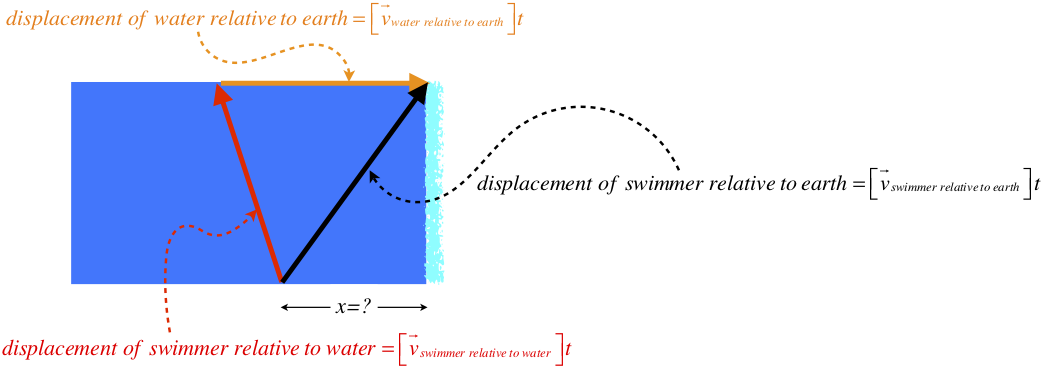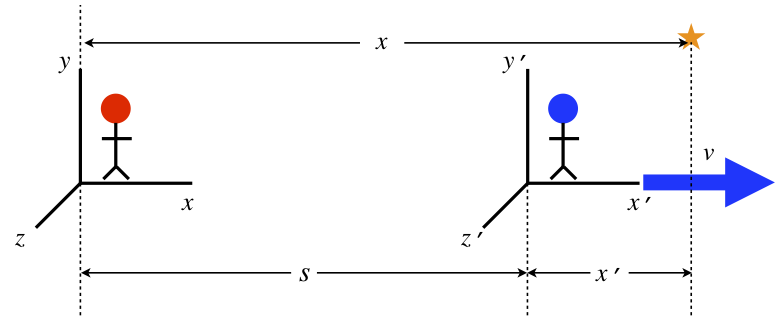$$\require{cancel}$$

# 1.8: Relative Motion

## Reference Frames

Our last topic for motion in multiple dimensions relates what different observers of the same motion measure for velocities. Let's start with the following simple example:

Ann and Bob are traveling on a train together. The train is traveling north at 60 mph, and while Bob remains in place on the train, Ann runs south through the dining car at 10 mph. Bob sees Ann traveling south at 10 mph, but Ann & Bob's mutual friend Chu, who is off the train and looking through the windows, sees Ann moving north at 50 mph. Both Bob and Chu are witnessing the same event, but they are doing so from do distinctly different perspectives, which we call reference frames. As a result of being in different reference frames, Bob and Chu make different measurements of Ann's velocity vector (they disagree on both the magnitude and direction of her motion).

If we can clearly describe how the two reference frames are related to one another, we can translate between Bob's measurements and Chu's by doing the proper mathematics. In the example above the mathematics is intuitive, but we will want a systematic way of doing it for more complicated situations, such as when the motions are not along the same line. It shouldn't be surprising that the way to do this is to bring vectors into the conversation.

## Relative Velocity Vectors

We begin by introducing some language. When an observer – who we will call "$$A$$" – in a given reference frame measures the velocity vector of an object (or another frame) – which we will call "$$B$$" – we express this vector in words and symbols in this way:

$"\text{velocity of } B \text{ relative to } A" \;\;\; \iff \;\;\; \overrightarrow v_{B\; rel \; A}$

Let's see if we can put the above example into this language. There are three entities here. Two are frames and on is a moving object. The moving object is Ann, and she is being observed by Bob, in the reference frame of the train, and Chu, in the reference frame of the earth. In the example, we expressed three different relative velocity vectors:

$"\text{velocity of Bob relative to Chu}" \iff \overrightarrow v_{b\; rel \; c} = \left(60mph\right) \widehat{north} \\ "\text{velocity of Ann relative to Bob}" \iff \overrightarrow v_{a\; rel \; b} = \left(10mph\right) \widehat{south} \\ "\text{velocity of Ann relative to Chu}" \iff \overrightarrow v_{a\; rel \; c} = \left(50mph\right) \widehat{north}$

Let's represent these three vectors as arrows beside each other in a diagram:

Figure 1.8.1 – Relative Velocity VectorsThe first thing we notice when we look closely at these is that our intuitive understanding of the original statement of the situation can be represented as a vector addition. Placing the the tail of the first vector at the head of the second vector, we find that the third vector can connect the open tail to the open head. In other words, we can express the result of the above example as a vector addition:

$\overrightarrow v_{a \; rel \; b} + \overrightarrow v_{b \; rel \; c} = \overrightarrow v_{a \; rel \; c}$

Note the ordering of the frames here is like a chain connecting Ann to Chu through Bob: Ann relative to Bob, then Bob relative to Chu, gives Ann relative to Chu. It turns out that this vector equation works not only when the velocities lie along a line, but also when they do not. For example, we can use the same vector equation if Ann were walking across the train (perpendicular to its motion.

There is one other feature of these relative velocity vectors that we will need, and that is reversing the perspective. In the case above, we have that Ann is moving 10 mph south relative to the Bob, but we can also talk about how Ann sees Bob moving relative to her. Bob starts off south of her, and as she runs by him, he ends up north of her. Therefore from Ann's perspective, Bob is moving north at 10 mph. So there is a simple way to alter a relative vector to reverse the perspective of reference frames: Switch the two frames in the subscript, and reverse the direction of the vector (i.e. multiply the original vector by –1). Here is a summary of these two rules:

Figure 1.8.2 – Summary of Relative Velocity RulesExample $$\PageIndex{1}$$

You stand on the bank of a river, contemplating swimming across, but the place where you hope to cross is close to a dangerous waterfall. When you look at the speed of the river, you estimate that it is about the same speed as you are able to swim. You realize that you can only swim so far in the cold water at this speed before your muscles shut down, and in still water you estimate that this distance is about $$100m$$. The width of the river is about $$80m$$.

1. Find the minimum distance that you must start upstream of the waterfall in order to not be swept over it.
2. If the river flows west-to-east and you start on its south shore, compute the direction in which you must swim in order to get safely across if you leave from the starting point computed in part (a).
Solution

a. Clearly to minimize the distance upstream that you need to start, you must swim with a component of your velocity relative to the river being upstream. The more you are able to turn yourself upstream, the less you will float downstream, and the closer you can start to the waterfall. But there is a limit to how far you can swim relative to the water, so your angle with the river must be such that when you reach your limit relative to the river, you reach the other side. The velocities are all constant and the time spans are all equal, so they are proportional to the displacements, which we can draw:We are given that the speed of the river relative to the earth is the same as the speed of the swimmer relative to the water, so we’ll call that quantity v, and the width of the river (which we know), we’ll call w. From the pythagorean theorem we can get the distance swum upstream against the current:

$distance \; swum \; against \; water = \sqrt{\left(vt \right)^2 - \left(w \right)^2} \nonumber$

The distance the water moves downstream relative to the earth is clearly vt, so the total distance the swimmer moves downstream is:

$x = vt - \sqrt{\left(vt \right)^2 - \left(w \right)^2} \nonumber$

But we actually know the value of vt, because it is the maximum distance that the swimmer can go in the water. Plugging in all the values therefore gives our answer:

$x = \left(100m \right) - \sqrt{\left(100m \right)^2 - \left(80m \right)^2} = \boxed{40m} \nonumber$

b. The angle is easy to determine, since we know the length of the displacement vector of the swimmer relative to the water and the width of the river:

$\cos\theta = \dfrac{w}{vt} = \dfrac{80m}{100m} \;\;\; \Rightarrow \;\;\; \theta = \boxed{37^o west \; of \; north} \nonumber$

## Galilean Transformation

Let's now consider two observers in difference reference frames that are moving at a constant speed relative to one another, which we will call $$v$$. We'll define the coordinate systems of these two observers such that their origins coincide at time $$t=0$$, and both observers agree on this starting time. Since the frames are moving relative to each other, this common origin only lasts for that one instant in time. We'll also define the coordinate systems such that they have common $$x$$, $$y$$, and $$z$$ axes when their origins coincide, and have their relative motion be along their common $$x$$-axis. We will label position coordinates and time measured by the frame moving in the $$+x$$-direction with a prime, to distinguish it from the other frame.

Suppose both observers record the motion of the same object. One observer gets equations of motion of this object for its three spatial coordinates $$\left(x, y, z \right)$$ as a function of time $$t$$, while the other observer gets equations of motion of the object for $$\left(x', y', z' \right)$$ as a function of time $$t'$$. The question we want to answer is, "Given what we know about how these frames are related to each other, what are the relations between the primed and unprimed coordinates?"

Let's start by noting that when the primed observer's origin has moved a distance $$s$$ relative to the unprimed observer's origin, the $$x$$-component of an object's position measured in the unprimed frame will be greater than the same component measured in the primed frame by that amount:

Figure 1.8.3 – Relating Coordinates of Reference FramesWe defined the frames so that their origins coincided when each of them measured the time to be zero, so the distance $$s$$ is simply equal to $$vt$$. The only difference in the two frames is in the $$x$$-direction, and the clocks are synchronized, so we have a complete translation of the two frames:

$\begin{array}{l} t' = t \\ x' = x-vt \\y'=y \\ z'=z \end{array}$

These are referred to as the Galilean transformation equations. They translate the coordinates of one frame into another that is moving relative to the first, with the restrictions indicated above regarding coinciding origins and so on. While this may not seem particularly interesting, keep in mind that these coordinates (when combined with cartesian unit vectors) compose the position vector, whose first derivative with respect to time is the velocity vector, etc. That is, every element of 3-dimensional kinematics – all the equations of motion of observed objects – can be translated into what they would be in another frame of reference through this transformation.

Example $$\PageIndex{2}$$

Ann and Bob are observers from different reference frames in relative motion, with all of the conditions necessary for their coordinate systems to be related by the Galilean transformation given above (Bob is in the primed frame, moving in the x-direction relative to Ann at a speed v). Ann observes a toy rocket moving in the y-direction with a speed $$u$$. Show that the velocity vector of this same rocket as measured by Bob is the same as would be obtained using the method of relative velocity vectors described in the previous section.

Solution

Let's start by computing the velocity vector of the ball according to Bob using the Galilean transformation. Taking the derivative of the position components with respect to time gives the components of the velocity vector seen by Bob, so substituting for $$t'$$ and $$x'$$ in the derivative gives:

$\left. \begin{array}{l} \dfrac{dx'}{dt'} = \dfrac{d\left( x-vt \right)}{dt} = \dfrac{dx}{dt} - v = -v \\ \dfrac{dy'}{dt'} = \dfrac{dy}{dt} = u \\ \dfrac{dz'}{dt'} = \dfrac{dz}{dt}=0 \end{array} \right\} \;\;\; \Rightarrow \;\;\; \overrightarrow u' = -v \widehat i + u \widehat j \nonumber$

Now let's use the tail-to-head relative velocity vector method from the previous section. The velocity of the rocket relative to Ann is $$u \widehat j$$, and the velocity of Bob relative to Ann is $$+v \widehat i$$. To get the velocity of the rocket relative to Bob, we need to form the "vector chain," which means we first need to get the velocity of Ann relative to Bob. Swapping the relative order requires only a minus sign, so doing this and putting together the vector chain gives:

$\left. \begin{array}{l} \text{velocity of rocket relative to Bob} = \overrightarrow u' \\ \text{velocity of rocket relative to Ann} = u \widehat j \\ \text{velocity of Ann relative to Bob} = -v \widehat i \end{array} \right\} \;\;\; \Rightarrow \;\;\; \overrightarrow u' = u \widehat j - v \widehat i \nonumber$